# Stats: One-Way ANOVA

A One-Way Analysis of Variance is a way to test the equality of three or more means at one time by using variances.

### Assumptions

• The populations from which the samples were obtained must be normally or approximately normally distributed.
• The samples must be independent.
• The variances of the populations must be equal.

### Hypotheses

The null hypothesis will be that all population means are equal, the alternative hypothesis is that at least one mean is different.

In the following, lower case letters apply to the individual samples and capital letters apply to the entire set collectively. That is, n is one of many sample sizes, but N is the total sample size.

### Grand Mean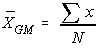The grand mean of a set of samples is the total of all the data values divided by the total sample size. This requires that you have all of the sample data available to you, which is usually the case, but not always. It turns out that all that is necessary to find perform a one-way analysis of variance are the number of samples, the sample means, the sample variances, and the sample sizes.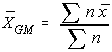Another way to find the grand mean is to find the weighted average of the sample means. The weight applied is the sample size.

### Total Variation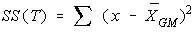The total variation (not variance) is comprised the sum of the squares of the differences of each mean with the grand mean.

There is the between group variation and the within group variation. The whole idea behind the analysis of variance is to compare the ratio of between group variance to within group variance. If the variance caused by the interaction between the samples is much larger when compared to the variance that appears within each group, then it is because the means aren't the same.

### Between Group Variation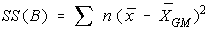The variation due to the interaction between the samples is denoted SS(B) for Sum of Squares Between groups. If the sample means are close to each other (and therefore the Grand Mean) this will be small. There are k samples involved with one data value for each sample (the sample mean), so there are k-1 degrees of freedom.

The variance due to the interaction between the samples is denoted MS(B) for Mean Square Between groups. This is the between group variation divided by its degrees of freedom. It is also denoted by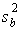.

### Within Group Variation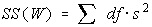The variation due to differences within individual samples, denoted SS(W) for Sum of Squares Within groups. Each sample is considered independently, no interaction between samples is involved. The degrees of freedom is equal to the sum of the individual degrees of freedom for each sample. Since each sample has degrees of freedom equal to one less than their sample sizes, and there are k samples, the total degrees of freedom is k less than the total sample size: df = N - k.

The variance due to the differences within individual samples is denoted MS(W) for Mean Square Within groups. This is the within group variation divided by its degrees of freedom. It is also denoted by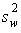. It is the weighted average of the variances (weighted with the degrees of freedom).

### F test statistic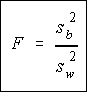Recall that a F variable is the ratio of two independent chi-square variables divided by their respective degrees of freedom. Also recall that the F test statistic is the ratio of two sample variances, well, it turns out that's exactly what we have here. The F test statistic is found by dividing the between group variance by the within group variance. The degrees of freedom for the numerator are the degrees of freedom for the between group (k-1) and the degrees of freedom for the denominator are the degrees of freedom for the within group (N-k).

### Summary Table

All of this sounds like a lot to remember, and it is. However, there is a table which makes things really nice.
 SS df MS F Between SS(B) k-1 SS(B) ----------- k-1 MS(B) -------------- MS(W) Within SS(W) N-k SS(W) ----------- N-k . Total SS(W) + SS(B) N-1 . .

Notice that each Mean Square is just the Sum of Squares divided by its degrees of freedom, and the F value is the ratio of the mean squares. Do not put the largest variance in the numerator, always divide the between variance by the within variance. If the between variance is smaller than the within variance, then the means are really close to each other and you will fail to reject the claim that they are all equal. The degrees of freedom of the F-test are in the same order they appear in the table (nifty, eh?).

### Decision Rule

The decision will be to reject the null hypothesis if the test statistic from the table is greater than the F critical value with k-1 numerator and N-k denominator degrees of freedom.

If the decision is to reject the null, then at least one of the means is different. However, the ANOVA does not tell you where the difference lies. For this, you need another test, either the Scheffe' or Tukey test.

### TI-82

Ok, now for the really good news. There's a program called ANOVA for the TI-82 calculator which will do all of the calculations and give you the values that go into the table for you. You must have the sample means, sample variances, and sample sizes to use the program. If you have the sum of squares, then it is much easier to finish the table by hand (this is what we'll do with the two-way analysis of variance)